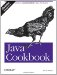# Recipe 5.10 Operating on a Series of Integers

#### Problem

You need to work on a range of integers.

#### Solution

For a contiguous set, use a for loop.

#### Discussion

To process a contiguous set of integers, Java provides a for loop. Loop control for the for loop is in three parts: initialize, test, and change. If the test part is initially false, the loop will never be executed, not even once.

 If the set of numbers is in an array or collection (see Chapter 7), use a "foreach" loop (see Recipe 8.2).

For discontinuous ranges of numbers, use a java.util.BitSet .

The following program demonstrates all of these techniques:

`import java.util.BitSet; /** Operations on series of numbers */ public class NumSeries {     public static void main(String[] args) {         // When you want an ordinal list of numbers, use a for loop         // starting at 1.         for (int i = 1; i <= months.length; i++)             System.out.println("Month # " + i);              // When you want a set of array indexes, use a for loop         // starting at 0.         for (int i = 0; i < months.length; i++)             System.out.println("Month " + months[i]);         // For a discontiguous set of integers, try a BitSet         // Create a BitSet and turn on a couple of bits.         BitSet b = new BitSet( );         b.set(0);    // January         b.set(3);    // April         // Presumably this would be somewhere else in the code.         for (int i = 0; i<months.length; i++) {             if (b.get(i))                 System.out.println("Month " + months[i] + " requested");         }     }     /** The names of the months. See Dates/Times chapter for a better way */     protected static String months[] = {         "January", "February", "March", "April",         "May", "June", "July", "August",         "September", "October", "November", "December"     }; }`Java Cookbook, Second Edition
ISBN: 0596007019
EAN: 2147483647
Year: 2003
Pages: 409
Authors: Ian F Darwin

Similar book on Amazon